# How to quickly insert Double strike or Blackboard bold symbols in Word equations

Word
Double strike or Blackboard bold is a typeface style that is often used for certain symbols in mathematical texts, in which certain lines of the symbol (usually vertical or near-vertical lines) are doubled. The symbols usually denote number sets (see some of usual symbols below).

To type the symbols in Double strike or Blackboard bold in the equation Microsoft Word (to insert equation into your text, click Alt+=), do one of the following:

• On the Equation tab, in the Symbols group, click the More button: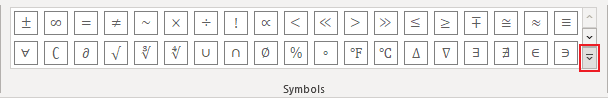In the top of list of symbols choose Scripts: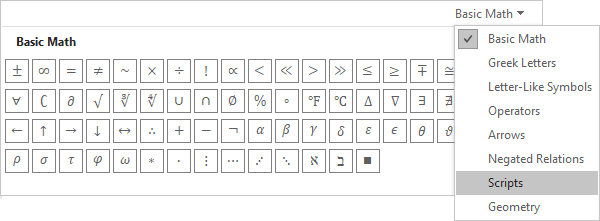In the Scripts list, in the Double-Struck group, choose the symbol you prefer: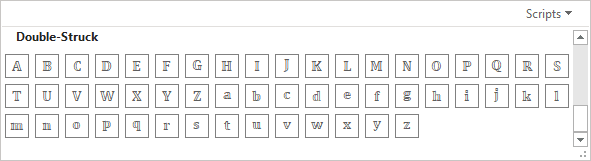• Type \double + capital or lowercase letter:

## Capital letters in the Double strike or Blackboard bold:

Symbol Code Description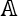\doubleA Represents the algebraic numbers or affine space in algebraic geometry.
Occasionally represents the algebraic numbers, the algebraic closure of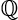(more commonly written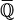or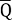), or the algebraic integers, an important subring of the algebraic numbers.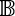\doubleB Sometimes represents a ball, a Boolean domain, or the Brauer group of a field.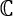\doubleC Represents the set of complex numbers.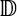\doubleD Represents the unit (open) disk in the complex plane (and by generalisationn may mean the n-dimensional ball) — for example as a model of the Hyperbolic plane. Occasionallymay mean the decimal fractions (see number) or split-complex numbers.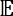\doubleE Represents the expected value of a random variable, or Euclidean space, or a field in a tower of fields, or the Eudoxus reals.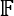\doubleF Represents a field. Often used for finite fields, with a subscript to indicate the order. Also represents a Hirzebruch surface or a free group, with a subset to indicate the number of generators (or generating set, if infinite).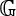\doubleG Represents a Grassmannian or a group, especially an algebraic group.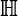\doubleH Represents the quaternions (the H stands for Hamilton), or the upper half-plane, or hyperbolic space, or hyperhomology of a complex.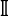\doubleI The closed unit interval or the ideal of polynomials vanishing on a subset. Occasionally the identity mapping on an algebraic structure, or an indicator function, or the set of imaginary numbers (i.e., the set of all real multiples of the imaginary unit, more commonly indicated i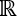).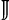\doubleJ Occasionally represents the set of irrational numbers, R\Q (\).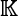\doubleK Represents a field, typically a scalar field. This is derived from the German word Körper, which is German for field (literally, "body"; cf. the French term corps). May also be used to denote a compact space.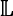\doubleL Represents the Lefschetz motive.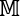\doubleM Sometimes represents the monster group. The set of all m-by-n matrices is sometimes denoted(m, n).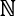\doubleN Blackboard bold capital N (for natural numbers set).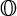\doubleO Represents the octonions.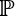\doubleP Represents projective space, the probability of an event, the prime numbers, a power set, the irrational numbers, or a forcing poset.\doubleQ Blackboard bold capital Q (for rational numbers set).\doubleR Represents the set of real numbers.represents the positive reals, whilerepresents the non-negative real numbers.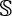\doubleS Represents a sphere, or the sphere spectrum, or occasionally the sedenions.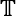\doubleT Represents the circle group, particularly the unit circle in the complex plane (andn the n-dimensional torus), or a Hecke algebra (Hecke denoted his operators as Tn or), or the tropical semi-ring, or twistor space.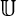\doubleU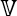\doubleV Represents a vector space or an affine variety generated by a set of polynomials.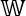\doubleW Occasionally represents the set of whole numbers (here in the sense of non-negative integers), which also are represented by0.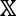\doubleX Occasionally used to denote an arbitrary metric space.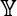\doubleY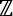\doubleZ Represents the set of integers.

## Some lowercase letters in the Double strike or Blackboard bold:

Symbol Code Description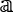\doublea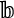\doubleb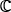\doublec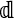\doubled May represent the differential symbol.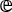\doublee Occasionally used for the mathematical constant e.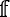\doublef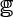\doubleg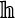\doubleh\doublei Occasionally used for the imaginary unit.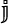\doublej

See also this tip in French: Comment insérer rapidement des symboles double barre ou blackboard gras dans l'équation Word.

If you have any questions or suggestions, please feel free to ask OfficeToolTips team.